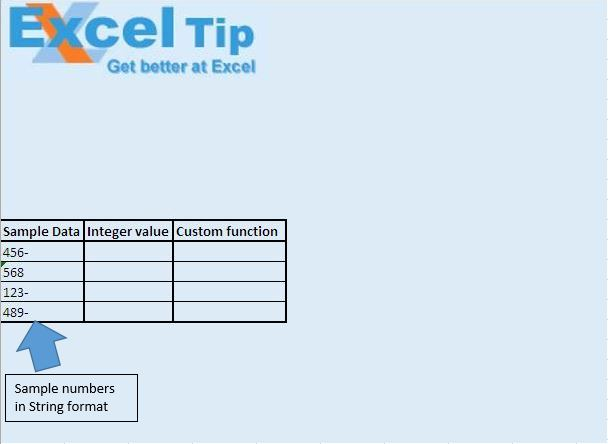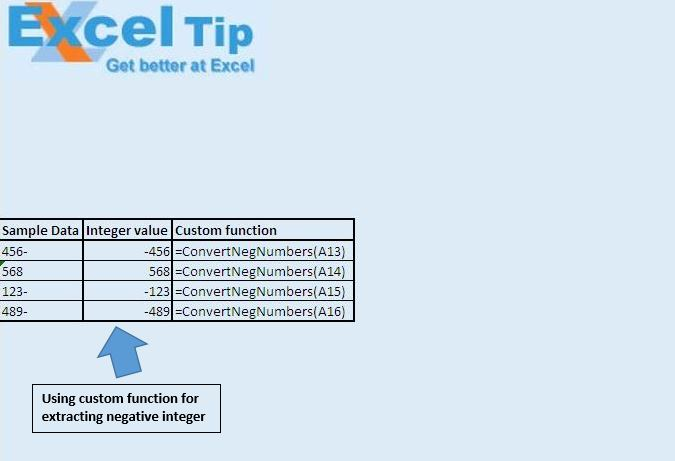# Convert negative values treated as text using VBA in Microsoft Excel

In this article, we will create a custom-function to convert negative strings to an integer.

Raw data for this article consists of strings followed by a minus sign (-).We want to convert these strings to integers.Logic explanation

In this article, we have created a custom-function ConvertNegNumbers to convert the string to integer. This function takes string value as input and returns integer as output.

Code explanation

Right(Var, 1) = "-"

The above code is used to check whether last character of the string is equal to minus sign (-).

ConvertNegNumbers = CInt("-" & Left(Var, Len(Var) – 1))

In above code, the left function is used to extract all the characters in the string, except the last one. The CInt function is used to convert string value to integer.

```Option Explicit

Function ConvertNegNumbers(Var As String)

'Checking whether last character in the string is minus(-)
If Right(Var, 1) = "-" Then

'Converting string value to negative integer
ConvertNegNumbers = CInt("-" & Left(Var, Len(Var) - 1))
Else

'Converting string value to integer
ConvertNegNumbers = CInt(Var)
End If

End Function
```